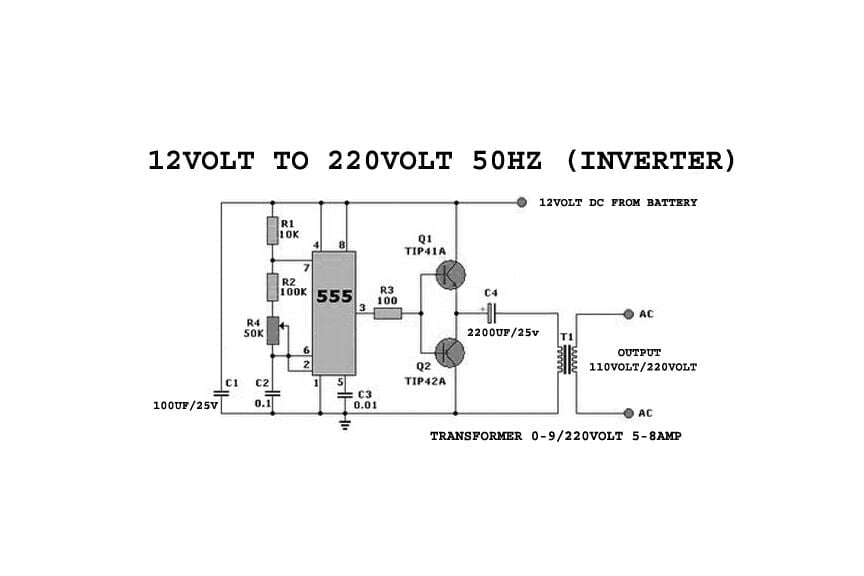# 12 V To 220 V Inverter Circuit 50 Hz

The heart Of this 12 V to 220 v inverter circuit diagram (50 hz) is  timer IC555, This Ic  is used as a switching pulse oscillator. IC 555 configured as Astable Multivibrator to give continuous switching pulse.

Two switching transistors TIP41A (NPN) and TIP42A (PNP) drives the transformer T1 according to the pulse input at the base. The transformer is 230V primary to 9V secondary but connected in reverse, So it can react as step up transformer.

We can apply +5V to +15V DC bias to this circuit and get 110V to 230V AC with 50Hz to 60Hz frequency. But output may not pure sine wave as the PWM inverter output, it gives only pulsated AC.

The output frequency of this Inverter circuit can be varied by varying VR1 resistor.

Use this formula to calculate the output AC supply and frequency, here R2 = R2+VR1 from given inverter circuit. Use heat sink for Transistors to avoid over heat.

1.5 v DC to 220 V AC inverter circuit Diagram with Mobile charger transformer. In this Project, we are going to make a very easy & simple low power inverter. Even this project can be made with scrap Continue Reading…..

## Parts List For 12 V To 220 V Inverter Circuit Diagram 50 Hz

• IC555 1pc
• Resistor 10k,100k, 100 ohm, all 1/4 watt, VR1 50k
• Capacitor 100uf/25v, 2200uf/25v, .1uf(104), .01uf(103)
• Transistor TIP41A, TIP42A
• Transformer 0-9/220Volt 5-8amp

## 12 V To 220 V Inverter Circuit Diagram 50 Hz